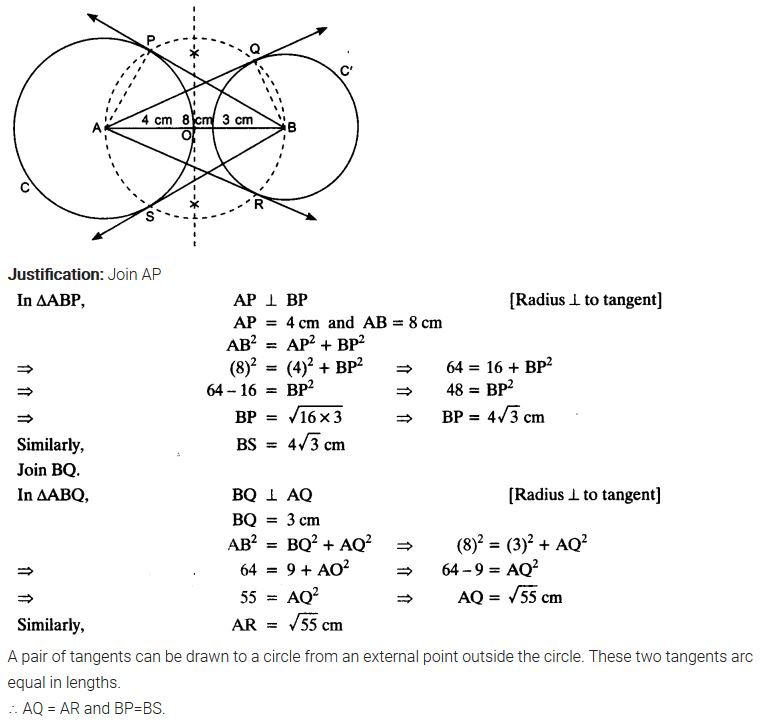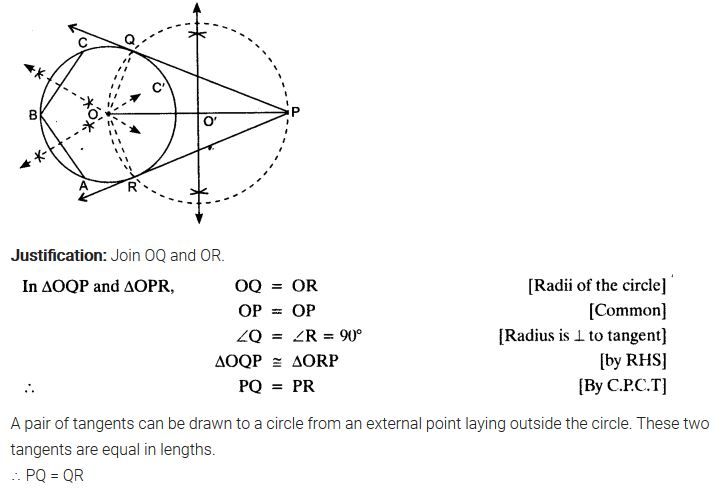ncert textbook

# NCERT Solutions for Class 10 Maths Chapter 11 Constructions Ex 11.2

Get Free NCERT Solutions for Class 10 Maths Chapter 11 Ex 11.2 PDF. Constructions Class 10 Maths NCERT Solutions are extremely helpful while doing your homework. Exercise 11.2 Class 10 Maths NCERT Solutions were prepared by Experienced LearnCBSE.in Teachers. Detailed answers of all the questions in Chapter 11 Maths Class 10 Constructions Exercise 11.2 provided in NCERT TextBook.NCERT Solutions for Class 10 Maths Chapter 11 Constructions Ex 11.2

NCERT Solutions for Class 10 Maths Chapter 11 Constructions Ex 11.2 are part of NCERT Solutions for Class 10 Maths. Here we have given NCERT Solutions for Class 10 Maths Chapter 11 Constructions Ex 11.2

In each of the following, give also the justification of the construction:

Question 1.
Draw a circle of radius 6 cm. From a point 10 cm away from its centre, construct the pair of tangents to the circle and measure their lengths.
Solution:Question 2.
Construct a tangent to a circle of radius 4 cm from a point on the concentric circle of radius 6 cm and measure its length. Also verify the measurement by actual calculation.
Solution:Question 3.
Draw a circle of radius 3 cm. Take two points P and Q on one of its extended diameter each at a distance of 7 cm from its centre. Draw tangents to the circle from these two points P and Q.
Solution:Question 4.
Draw a pair of tangents to a circle of radius 5 cm which are inclined to each other at an angle of 60°.
Solution:Question 5.
Draw a line segment AB of length 8 cm. Taking A as centre, draw a circle of radius 4 cm and taking B as centre, draw another circle of radius 3 cm. Construct tangents to each circle from the centre of the other circle.
Solution:Question 6.
Let ABC be a right triangle in which AB = 6 cm, BC = 8 cm and ∠B = 90°. BD is the perpendicular Burn B on AC. The circle through B, C, D is drawn. Construct the tangents from A to this circle.
Solution:Question 7.
Draw a circle with the help of a bangle. Take a point outside the circle. Construct the pair of tangents from this point to the circle.
Solution: